## Divide Polynomials Part I

### Learning Outcome

• Multiply and divide polynomials

## Divide a Polynomial by a Binomial

Dividing a polynomial by a monomial can be handled by dividing each term in the polynomial separately. This cannot be done when the divisor has more than one term. However, the process of long division can be very helpful with polynomials.

Recall how you can use long division to divide two whole numbers, say $900$ divided by $37$.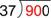First, you would think about how many $37s$ are in $90$, as $9$ is too small. (Note: you could also think, how many $40s$ are there in $90$.)

There are two $37s$ in $90$, so write $2$ above the last digit of $90$. Two $37s$ is $74$; write that product below the $90$.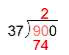Subtract: $90–74$ is $16$. (If the result is larger than the divisor, $37$, then you need to use a larger number for the quotient.)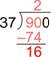Bring down the next digit $(0)$ and consider how many $37s$ are in $160$.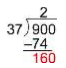There are four $37s$ in $160$, so write the $4$ next to the two in the quotient. Four $37s$ is $148$; write that product below the $160$.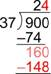Subtract: $160–148$ is $12$. This is less than $37$ so the $4$ is correct. Since there are no more digits in the dividend to bring down, you are done.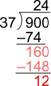The final answer is $24$ R$12$, or $24\frac{12}{37}$. You can check this by multiplying the quotient (without the remainder) by the divisor, and then adding in the remainder. The result should be the dividend:

$24\cdot37+12=888+12=900$

To divide polynomials, use the same process. This example shows how to do this when dividing by a binomial.

### Example

Divide: $\frac{\left(x^{2}–4x–12\right)}{\left(x+2\right)}$

In the following video, we show another example of dividing a degree two trinomial by a degree one binomial.

Let us try another example. In this example, a term is “missing” from the dividend.

### Example

Divide: $\frac{\left(x^{3}–6x–10\right)}{\left(x–3\right)}$

In the video that follows, we show another example of dividing a degree three trinomial by a binomial. Note the “missing” term and how we work with it.

The process above works for dividing any polynomials no matter how many terms are in the divisor or the dividend. The main things to remember are:

• When subtracting, be sure to subtract the whole expression, not just the first term. This is very easy to forget, so be careful!
• Stop when the degree of the remainder is less than the degree of the dividend or when you have brought down all the terms in the dividend and your quotient extends to the right edge of the dividend.

In the next video, we present one more example of polynomial long division.

## Summary

Dividing polynomials by polynomials of more than one term can be done using a process very much like long division of whole numbers. You must be careful to subtract entire expressions, not just the first term. Stop when the degree of the remainder is less than the degree of the divisor. The remainder can be written using R notation or as a fraction added to the quotient with the remainder in the numerator and the divisor in the denominator.